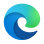Version 1.0, Genesis, is now available.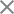# Spherical phase lens. Diffraction propagation.

In summary, the “thin element approximation” approach and phase masks enable the realistic modeling of various optical elements, and by manipulating the phase shifts, one can accurately simulate the behavior of lenses and other optical components within the 3DOptics software.”

Different kinds of masks can be prepared. To model a spherical lens with a specified focal length macked from the glass with a specified refractive index n_{lens} , a spherical phase mask can be prepared on the base of the “lens-maker” equation.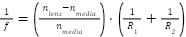Assuming R_2 = \infin (plano-convex or a plano-concave lens), and n_{media} = 1 radius R_1 can be found: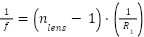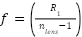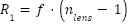The Sag of the lens can be calculated by using the sphere equation with radius R_1 as function of radial coordinate r = \sqrt{x^2 + y^2} , where x and y are cartesian coordinates of the specific point on the mask plane (Figure 1).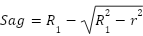Phase shift \Delta\varphi introduced by the Sag is: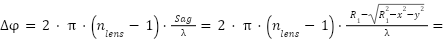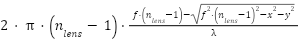where \lambda is a wavelength of the incident wave.

The mask should be wrapped with the range 2\pi before using it in 3DOptics.

The Huygens integral, derived from Huygens’ principle, is a mathematical representation used to calculate the diffraction pattern resulting from a given diffracting object. It involves integrating the contributions of the secondary wavelets across the entire diffracting aperture to determine the resulting wavefront. The Huygens integral is a powerful tool in wave optics, enabling the prediction and analysis of diffraction patterns in various situations.

GPU-based implementations of Huygens’ integral can greatly accelerate the simulation of diffraction patterns, enabling researchers to study complex scenarios with higher accuracy and faster turnaround times. This can be particularly beneficial in fields such as optics, where the analysis of intricate diffraction phenomena plays a crucial role in designing optical systems and devices.

The integration of GPU processors in implementing Huygens’ integral offers the potential for significant advancements in simulating and analyzing diffraction phenomena. By leveraging the parallel computing capabilities of GPUs, researchers can explore more complex scenarios, improve accuracy, and accelerate the understanding of wave optics phenomena.

We have implemented a GPU-based numerical solution of a modified scalar Huygens integral (Equation 2, Ref. 1) for the near field without any assumptions. The computation time for the present GPU configuration is 3 seconds for 500X500 sampling in the object plane and 500X500 sampling in the image plane. Amplitude and phase masks can be used as elements of the optical scheme, including spherical-phase masks, amplitude and phase grating masks, and phase holograms (thin film approximation). We are now working on implementing a 4-f model based on spherical phase elements for modeling Fourier optics problems.

Ref. 1. Saleh, B.E.A. (2007). Fundamentals of Photonics (2nd ed.). Wiley.## 3DOptix worksonly on desktop!

Please go to 3doptix.com on a
desktop device, using the
Chrome or Edge browser
•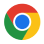•